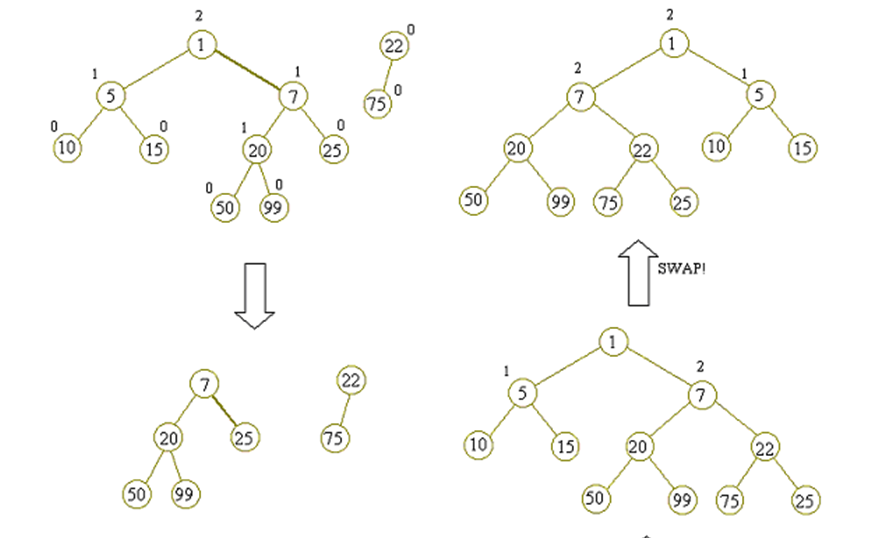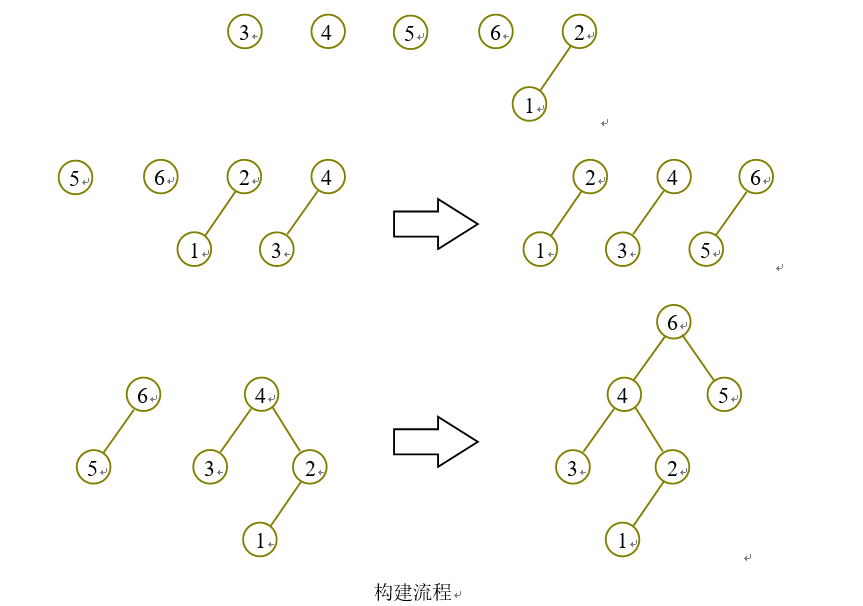# 左偏树(Leftist Tree)是一种可并堆的实现。

[性质1] 节点的键值小于或等于它的左右子节点的键值。即key(i)≤key(parent(i))

##### [性质2] 节点的左子节点的距离不小于右子节点的距离。即dist(left(i))≥dist(right(i))

[性质3] 节点的距离等于它的右子节点的距离加1。即dist(i)=dist(right(i))+1。

[引理1] 若左偏树的距离为一定值，则节点数最少的左偏树是完全二叉树。 证明：由性质2可知，当且仅当对于一棵左偏树中的每个节点i，都有dist(left(i))=dist(right(i))时，该左偏树的节点数最少。显然具有这样性质的二叉树是完全二叉树。

[定理1] 若一棵左偏树的距离为k，则这棵左偏树至少有2^(k+1)-1个节点。 证明：由引理1可知，当这样的左偏树节点数最少的时候，是一棵完全二叉树。距离为k的完全二叉树高度也为k，节点数为2^(k+1)-1，所以距离为k的左偏树至少有2k＋1-1个节点。

[性质4] 一棵N个节点的左偏树距离最多为O(log(N+1)-1)。

## 左偏树的合并

C ← Merge(A,B) 把A,B两棵左偏树合并，返回一棵新的左偏树C，包含A和B中的所有元素。在本文中，一棵左偏树用它的根节点的指针表示。

right(A) ← Merge(right(A),B)## 左偏树的构建``````#include <algorithm> //STL通用算法
#include <cmath> //定义数学函数
#include <cstdio> //定义输入/输出函数
#include <iostream> //数据流输入/输出
#include <cstring> //字符串处理
#include <string> //字符串类
#include <ctime> //定义关于时间的函数
#define itn int
#define fro for
#define ll long long
#define reg register
#define inf 1234567890
/*#include <bitset> //STL位集容器
#include <cstype> //字符处理
#include <cerrno> //定义错误码
#include <complex> //复数类
#include <clocale> //定义本地化函数
#include <cstdlib> //定义杂项函数及内存分配函数
#include <deque> //STL双端队列容器
#include <exception> //异常处理类
#include <fstream> //文件输入/输出
#include <functional> //STL定义运算函数(代替运算符)
#include <limits> //定义各种数据类型最值常量
#include <list> //STL线性列表容器
#include <map> //STL映射容器
#include <iomanip> //参数化输入/输出
#include <ios> //基本输入/输出支持
#include <iosfwd> //输入/输出系统使用的前置声明
#include <istream> //基本输入流
#include <ostream> //基本输出流
#include <queue> //STL队列容器
#include <set> //STL集合容器
#include <sstream> //基于字符串的流
#include <stack> //STL堆栈容器
#include <stdexcept> //标准异常类
#include <streambuf> //底层输入/输出支持
#include <utility> //STL通用模板类
#include <vector> //STL动态数组容器
#include <cwchar.h>//宽字符处理及输入/输出
#include <cwctype.h> //宽字符分类*/

using namespace std;

int ans;

int max(int x,int y){return x>y?x:y;}

int min(int x,int y){return x<y?x:y;}

void swap(int &x,int &y){int d=x;x=y;y=d;}

int read()
{
int x=0,f=1;char ch=getchar();
while (ch<'0' || ch>'9'){if (ch=='-')f=-1;ch=getchar();}
while ('0'<=ch && ch<='9'){x=x*10+(ch^48);ch=getchar();}
return x*f;
}

void write(int x)
{
int buf;
if (x<0) putchar('-'),x=-x;
buf=0;
while (x) buf[++buf]=x%10,x/=10;
if (!buf) buf=1,buf=0;
while (buf) putchar('0'+buf[buf--]);
}

int fa,dist,Left,Right,key;

int find(int x)
{
return fa[x]?find(fa[x]):x;
}

int merge(int x,int y)
{
if (x==0) return y;
if (y==0) return x;
if (key[y]<key[x]||(key[x]==key[y]&&x>y)) swap(x,y);
int &l=Left[x],&r=Right[x];
r=merge(r,y);
fa[r]=x;
if (dist[r]>dist[l]) swap(l,r);
dist[x]=dist[r]+1;
}

void Delete(int x)
{
key[x]=-1;
fa[Right[x]]=fa[Left[x]]=0;
merge(Left[x],Right[x]);
}

int main(){
int n=read(),m=read();
dist=-1;
for (int i=1;i<=n;i++) key[i]=read();
while (233333&&m--)
{
int q=read();
if (q==1)
{
int x=read(),y=read();
if (key[x]==-1||key[y]==-1) continue;
x=find(x),y=find(y);
if (x!=y) merge(x,y);
}
if (q==2)
{
int x=read();
if (key[x]==-1) printf("-1\n");
else
{
x=find(x);
printf("%d\n",key[x]);
Delete(x);
}
}
}
return 0;
}``````